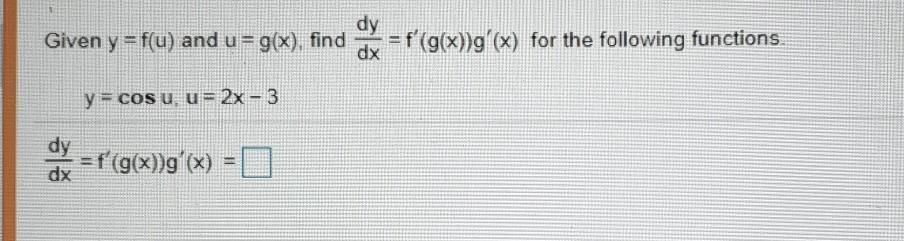### Create an Account

Home / Questions / dy Given y=f(u) and u = g(x), find = f(g(x))g'(x) for the following functions dx = ') y = ...

# dy Given y=f(u) and u = g(x), find = f(g(x))g'(x) for the following functions dx = ') y = cos u. u= 2x - 3 dy =f(g(x))g'(x) = dx

dy Given y=f(u) and u = g(x), find = f(g(x))g'(x) for the following functions dx = ') y = cos u. u= 2x - 3 dy =f(g(x))g'(x) = dxMay 05 2021 View more View LessSubscribe To Get Solution